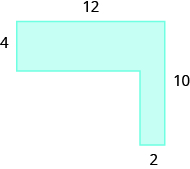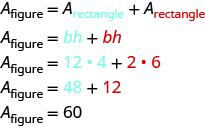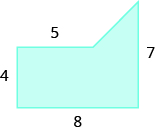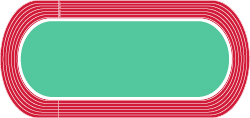## Finding the Area of Irregular Figures

### Learning Outcomes

• Find the area of irregular figures

So far, we have found area for rectangles, triangles, trapezoids, and circles. An irregular figure is a figure that is not a standard geometric shape. Its area cannot be calculated using any of the standard area formulas. But some irregular figures are made up of two or more standard geometric shapes. To find the area of one of these irregular figures, we can split it into figures whose formulas we know and then add the areas of the figures.

### example

Find the area of the shaded region.Solution
The given figure is irregular, but we can break it into two rectangles. The area of the shaded region will be the sum of the areas of both rectangles.The blue rectangle has a width of $12$ and a length of $4$. The red rectangle has a width of $2$, but its length is not labeled. The right side of the figure is the length of the red rectangle plus the length of the blue rectangle. Since the right side of the blue rectangle is $4$ units long, the length of the red rectangle must be $6$ units.The area of the figure is $60$ square units.
Is there another way to split this figure into two rectangles? Try it, and make sure you get the same area.

### try it

The following video gives another example of how to find the area of an “L” shaped polygon using the dimensions of two rectangles.

### example

Find the area of the shaded region.### example

A high school track is shaped like a rectangle with a semi-circle (half a circle) on each end. The rectangle has length $105$ meters and width $68$ meters. Find the area enclosed by the track. Round your answer to the nearest hundredth.### try it

The next video example is similar to the previous example, but the object for which we find area only contains one semi-circle.

## Contribute!

Did you have an idea for improving this content? We’d love your input.